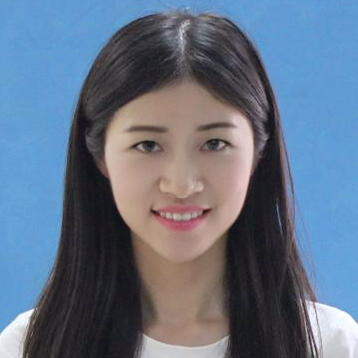﻿ 韩迪-西北大学数学学院

# 教师简介• 姓名：韩迪
• 部门：基础数学系
• 职称：副教授
• 荣誉：硕士生导师
• 电子邮件：dhan@nwu.edu.cn
• 研究方向：数论及其应用

2011.9--2012.7  西北大学数学系    基础数学专业  硕士研究生
2012.9--2016.7  西北大学数学学院  基础数学专业  博士研究生

2020.10--至今   西北大学数学学院  副教授

• 1.主要课题

• 1. 主持国家自然科学基金青年项目，Dirichlet L-函数、Gauss和及相关问题研究(11701447)，2018.1-2020.12.
2. 主持陕西省自然科学基础研究项目，指数和及其应用(2017JQ1002), 2017.1-2018.12.
3. 主持西北大学自主创新项目, 指数和与特征和的混合均值研究(YZZ13071), 2014.1-2015.6.
4. 参与国家自然科学基金面上项目, L-函数、大值特征和及相关问题研究(11471258), 2015.1-2018.12.
5. 参与国家自然科学基金青年项目, 密码函数的复杂性分析与构造(61202437), 2013.1-2015.12.
6. 参与陕西省自然科学基金项目, 特征和及其应用(2014JM1001), 2014.1-2015.12.
7. 参与西北大学自主创新项目, 数列、指数和及特征和的性质研究(YZZ12062), 2013.1-2014.6.

2.主要成果

1. Han Di* and Zhang Wenpeng, On the existence of some special primitive roots mod p, Bull. Math. Soc. Sci. Math. Roumanie(SCI), 58(2015), No.1, 59-66.
2. Han Di* and Zhang Wenpeng, A new mean value related to D.H.Lehmer's problem and Kloosterman sums, Bulletin of the Korean Mathematical Society(SCI), 52(2015), No.1, 35-43.
3. Han Di*, Some special primes and square sums, Quaestiones Mathematicae(SCI), 38(2015), Issue 1, 57-62.
4. Han Di* and Wang Tingting, On the Dedekind sums and two-term exponential, Chinese Annals of Mathematics, Series B(SCI), 36(2015), No.4, 603-612.
5. Han Di* and Zhang Wenpeng, The Ternary Exponential Diophantine Equation Concerning Pythagorean Triplets, Quaestiones Mathematicae (SCI), 38(2015), No.4, 483-494.
6. Han Di* and Zhang Wenpeng, Some new identities involving Dedekind sums and the Ramanujan sum, Ramanujan Journal(SCI), 35(2014), 253-262.
7. Han Di* and Guan Wenji, A note on the Diophantine equation (x(p)-1)/(x-1)=p(e)y(q), Bulletin Mathematique de la Societe Des Sciences Mathematiques de Roumanie(SCI),  57(2014), No.1, 35-43.
8. Han Di*, A hybrid mean value involving two-term exponential sums and  polynomial character sums, Czechoslovak Mathematical Journal(SCI),  64(139)(2014),  No.1, 53-62.
9. Han Di* and Zhang Wenpeng, Upper bound estimate of character sums  over D. H. Lehmer's numbers, Journal of Inequalities and Applications(SCI), 2013:392 (20, August 2013).
10. Han Di* and Zhang Wenpeng, On the Hybrid Mean Value Involving Kloosterman Sums and Sums Analogous to Dedekind Sums, Abstract and Applied Analysis(SCI), Article ID: 760505,  2013.
11. Zhang Wenpeng and Han Di*, A new identity involving the classical Kloosterman sums and 2-dimensional Kloosterman sums, International Journal of Number Theory(SCI), 12(2016), No.1, 111-119.
12. Zhang Wenpeng and Han Di*, On the sixth power mean of the two-term exponential sums, Journal of Number Theory(SCI), 136(2014), 403-413.
13. Zhang Wenpeng and Han Di, A hybrid mean value of Dedekind sums and Kloosterman sums, Journal of Number Theory(SCI), 147(2015), 861-870.
14. Chen Guohui and Han Di*, A new sums and its reciprocity theorem,Miskolc Mathematical Notes(SCI), 17(2017), No.2, 811-816.
15. Wang Xiaohan and Han Di*, Some identities related to Dedekind sums and the Chebyshev polynomials, International Journal of Applied Mathematics and Statistics(EI), 51(2013), No.21, 334-339.
16. Liu Baoli and Han Di*, On the Fermat prime and the mean value of L-functions, International Journal of Applied Mathematics and Statistics(EI), 49(2013), No.19,152-156.
17. Feng Qiang and Han Di*, On the Diophantine system  and   for   is a prime, International Journal of Applied Mathematics and Statistics, 52(2014), No.7, 65-73.
18. 韩迪, 一些特殊整数的分拆问题, 数学进展, 43(2014), No.2, 263-266.
19. 韩迪, 关于特定幸福立方数列, 郑州大学学报, 44(2012), No.3, 20-21.
20. 杜先存, 韩迪*, 关于三项指数和的四次均值问题, 西北大学学报（自然科学版）, 43(2013), No.4, 541-544.

21. Han Di and Lv Xingxing, On the Chebyshev polynomials and some of their new identities, Advances in Difference Equations, 2020:86, 1-8. (SCI)
22. Han Di and Zhang Wenpeng, A certain mean square value involving Dirichlet L-functions, Mathematics, 2020, 8, 948, 1-11. (SCI)
23. Han Di and Zhang Wenpeng, On the Chebyshev polynomials and some of their reciprocal sums, Symmetry 2020, 12, 704, 1-9. (SCI)

3.获奖情况

1. 获2013年博士研究生国家奖学金
2. 博士毕业论文“Dirichlet L-函数、Gauss和及其应用”被评为西北大学优秀博士学位论文

3. 获西北大学第十二届青年教师讲课比赛一等奖（理工组）

4. 2019年西北大学教学奖
5. 获西北大学数学学院青年教师多媒体课件设计竞赛二等奖
6. 获西北大学课堂教学创新大赛一等奖（理工组）
7. 指导学生参加全国大学生数学建模竞赛获全国一等奖1项